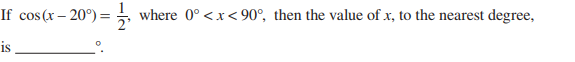# Thread: How do I solve cos(X-20degrees)= 1/2 ?

1. ## How do I solve cos(X-20degrees)= 1/2 ?This is the question. Please help!

2. ## Re: How do I solve cos(X-20degrees)= 1/2 ?Originally Posted by SeirinabaThis is the question. Please help!
$\cos\left(x-\frac{\pi}{9}\right)=\frac{1}{2}$ which implies that $\left(x-\frac{\pi}{9}\right)=\frac{\pi}{6}$.
Therefore $x=\frac{\pi}{6}+\frac{\pi}{9}=\frac{5\pi}{18}$

3. ## Re: How do I solve cos(X-20degrees)= 1/2 ?

how did you turn 1/2 into π/6?

4. ## Re: How do I solve cos(X-20degrees)= 1/2 ?Originally Posted by Seirinabahow did you turn 1/2 into π/6?
As a matter of fact $\frac{1}{2}$ does not turn into $\frac{\pi}{6}$

Because I do not know what a degree is, I chose to do mathematics using numbers.
I just know and you must learn that if $\cos(\theta)=\frac{1}{2}$ then $\theta=\pm\frac{\pi}{6}$.
That is just a fact of the measure of angles.

5. ## Re: How do I solve cos(X-20degrees)= 1/2 ?Originally Posted by SeirinabaThis is the question. Please help!
$\cos \frac{\pi }{3}=\frac{1}{2}$
$x=\frac{\pi }{3}+\frac{\pi }{9}=\frac{4 \pi }{9}$
circle, sinusoidal function, triganometry